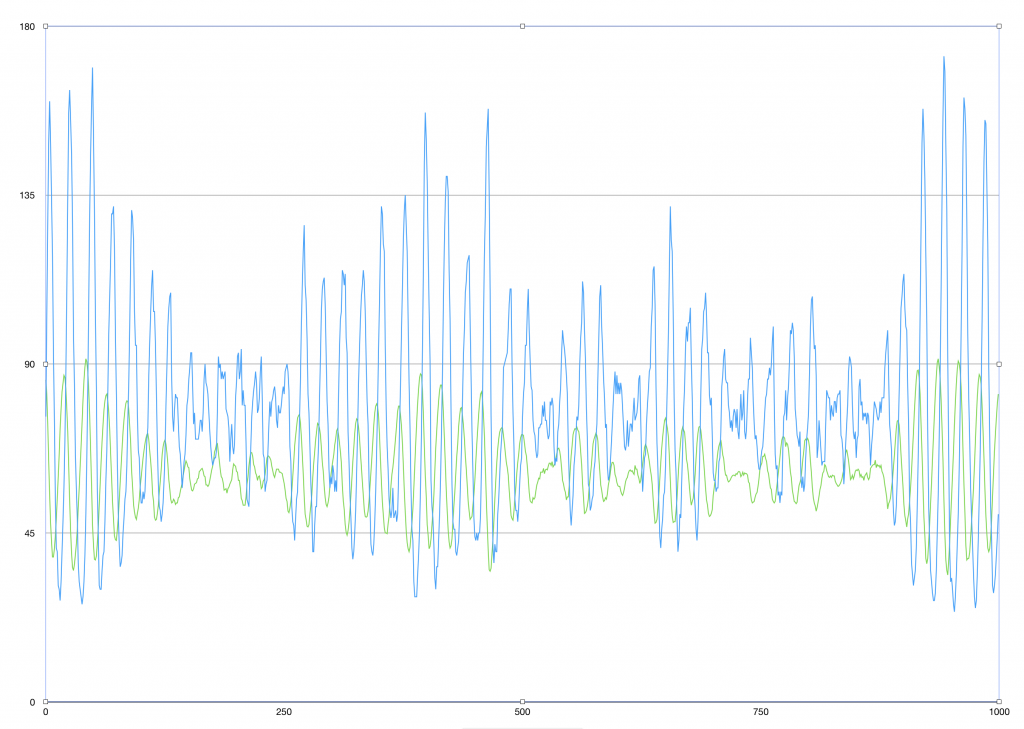Introductory Activity:

Review the code you wrote last time and decide what you need to finish today.

The following is a good goal for the end of today. Results from the simulation are found below the code.

Moose Class:

`class Moose {    var mooseAgeMaturity = 5 // age a moose needs to be to reproduce    var mooseLongevityAge = 100 // number of years a moose will live naturally    var age = 0    var alive = true // moose is alive or dead    var vegetationNeededToSurvive = 0.01 // each moose needs to eat 1% worth of the islands vegetation per year    var mooseBirthRate = 0.75 // each moose will create 0.75 moose each year once it’s old enough to mate    var starvation = 0    var environment: Environment    init(in environment: Environment, age: Int) {        mooseAgeMaturity = 5        let deltaLife = Int.random(in: -6 ... 6)  // randomize the longevity of a moose - simulation not very sensitive to this feature        mooseLongevityAge = 20 + deltaLife        self.age = age        alive = true        vegetationNeededToSurvive = 0.002  //  fraction of the region's vegetation a single moose eats per year        mooseBirthRate = 0.75  // fraction of a moose another moose gives birth to, assuming it is mature enough        starvation = 0    //  counter to keep track of how many years a moose has gone without sufficient food        self.environment = environment    }    func canEat() -> Bool {        //  current vegetation cover in the region is a fraction from 0.0 to 1.0        //  randomize the probability that a moose finds itself in the vegetated fraction of the region and can eat sufficiently for a year        let randomNumber = Double.random(in: 0.0 ... 1.0)        if randomNumber > environment.vegCover {            return false        }        return true    }        func liveForYear() {                // If a moose doesn’t find food for two years in a row, it dies        // If a moose survives until mooseLongevityAge, it dies                if canEat() {            age += 1            environment.vegCover -= vegetationNeededToSurvive        } else {            starvation += 1        }        if starvation >= 2 { alive = false }        if age >= mooseLongevityAge { alive = false }            }   }`

Environment Class:

`class Environment {    var vegCover = 1.0    let vegatationRegrowthRate = 0.1  // rate at which vegetation regrows each year    var mooseArray = [Moose]()  //  create an empty array of moose        func updateVegetation() {        //  grow vegetation cover for a year        vegCover += vegatationRegrowthRate        if vegCover > 1.0 { vegCover = 1.0 }    }        //  update simulation population    func updateMoosePopulation() {                var newMooseArray = [Moose]()        var newMooseBabies = 0.0        //  check and see if there are any dead moose, of so, remove them from the mooseArray        for i in mooseArray.indices {            let thisMoose = mooseArray[i]            //  each moose experiences a year            thisMoose.liveForYear()                       //  determine how many new moose will be born this year            if thisMoose.alive {                newMooseArray.append(thisMoose)                if thisMoose.age >= thisMoose.mooseAgeMaturity {                    newMooseBabies += thisMoose.mooseBirthRate                }            }        }                //  have moose reproduce        let numberOfBabies = Int(newMooseBabies)        //  add new moose babies to the herd        for _ in 0 ..< numberOfBabies {            newMooseArray.append(Moose(in: self, age: 0))        }                mooseArray = newMooseArray    }}`

Simulation Class:

`class Simulation {    let numberOfInitialMoose = 80    let simulationLength = 1000 //  number of years for the simulation    var year = 0 // year of the simulation    var environment = Environment()    var numberOfMooseInSimulation: Int { // a computed property that return the current number of moose in herd        return environment.mooseArray.count    }    init() {  // initialize the simulation        environment = Environment()        for _ in 0 ..< numberOfInitialMoose {            let initialAge = Int.random(in: 1 ... 30)            environment.mooseArray.append(Moose(in: environment, age: initialAge))        }    }    //  Simulation of Isle Royale Moose    func simulateYear() {        environment.updateVegetation()        environment.updateMoosePopulation()    }        func runSimulation() {        for year in 0 ..< simulationLength {            simulateYear()            print("\(year), \(numberOfMooseInSimulation), \(environment.vegCover * 100.0)")        }    }    }`

Main Program:

The main program is short and sweet. Create a single instance of the Simulation class and call the runSimulation method on the instance.

`var sim001 = Simulation()sim001.runSimulation()`

Simulation Results:Results from 1000-year simulation of moose-vegetation model. Blue: Moose Population; Green: Vegetation Fraction

Summary Activity:

Discuss the results of the 1000-year simulation:

Questions:

• How realistic is the model?
• Does the model match the observations in any ways (not enough moose; ~25 cycle of moose population expansion and decay)
• Ideas on how we can make the simulation match observations more accurately (decrease amount moose eats per year? increase moose birth rates? increase vegetation growth rates

Homework?

What kinds of systematic experiments would you like to run with our model?

Spend the last 5 minutes dividing up simulation experiments for each student to perform. Use the questions above to guide the experiments. Have the students write clear hypotheses before leaving class and performing the experiments.